# LOG#179. Extra dimensions: Kepler 3rd law.Hi, everyone! Long time since the last time I wrote here. I am sorry. Life is complex and complicated…But I know you are there, dear followers.

Today, Kepler motion and Kepler third law with extra dimensions! Are you prepared?

Let me review some basics. You learned from high school or college Newton gravitational law

(1)And with a bit of mathematical and physical tricks you get

(2)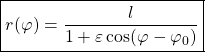That is Kepler 1st law. You also get, via angular momentum conservation, Kepler 2nd law

(3)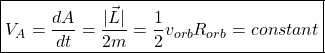And one of the main topics of this post, the famous Kepler 3rd law (a type of harmony in celestial motion via power laws:

(4)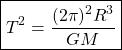Generally,is the total mass of the kepler (two-body!) motion. Therefore, there is a power law in the sexy (have you experienced it? Do you have a companion star to revolve around of it?) two body keplerian motion under the gravitational (universal!) force.

The keplerian 3rd law for circular orbits (for elliptical motion, the most general motion, given by the 1st law, you need a hidden symmetry and vector to get the full solution, but that will be the topic of a future post on hidden symmetries) can be obtained from the gravitational law of Newton and centripetal force:

(5)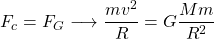Since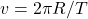, you get after simple algebra

(6)Furthermore, you can get from this equations and laws the orbital velocity, the orbital angular velocity, and the orbital frequency:

(7)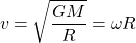(8)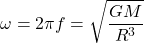(9)In 3d space, you can also relate all these magnitudes with density of the revolving objects, if you plug, with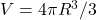, you will get

(10)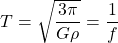(11)(12)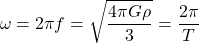Even more interestingly, and lot of times lost in books of physics, you can relate orbital velocity with period and mass or density combining the above equations from universal gravitation with the Kepler 3rd law. Simple algebra again provides:

(13)that withtransforms as

(14)so

(15)The missing equation (in books) relates orbital velocity to frequency or period directly:

(16)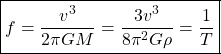and it is useful for Astronomy, binary systems with some more general treatment (have you ever known what the function of mass is? The function of mass for binary systems is closely related to all this stuff…). Before extending these equations to arbitrary spacetime dimensions and forces, let me talk about the well know harmonic oscillator. If the force that makes planets to orbit were the Hooke law, the periods of all the planets will be the same and the world very different!!!! Mimicking the previous arguments, for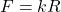, you get

(17)and thus, EVERY planet would have a period

(18)as you can see,does NOT depend on, the orbital radius. What a strange Universe would it be! The orbital velocity can also be calculated:

(19)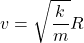The dark matter problem would be “solved” if the law for gravity at long scales were a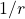power law. As you can guess from the same trickery

(20)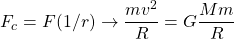and it implies

(21)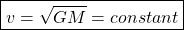So, in this case we obtain

(22)Now, as promised, I will generalize these arguments for kepler motion in extra dimensions! You can mimic it as exercise for the Coulomb force (indeed I have already talked about that when I wrote on the Bohr model and its quantization). With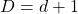spacetime dimensions, the universal law for gravity and its circular orbits come from

(23)For the orbital velocity you get in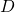-spacetime,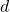-space dimensions,

(24)or equivalently

(25)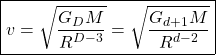For circular orbits, again, you can obtain the generalized kepler 3rd law for extra dimensions, as follows

(26)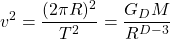and from simple mathematical manipulations you deduce

(27)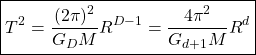From this, one of our main results, you can also get the frequencies and angular velocities

(28)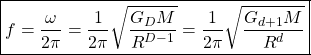Using the generalized third law of Kepler with extra dimensions (and circular orbits, don’t forget our hypotheses), you can relate orbital velocity with mass and frequency (or period) directly for any space-time dimension. Algebraic operations (check it!) provide the following main results:

(29)(30)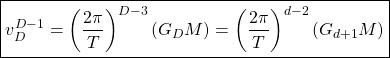And for the frequencies:

(31)The above beautiful formulae are valid for gravitational circular motion in any space with d=2,3,4,…Or D=3,4,5,…space-time. They are laws for keplerian motion in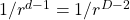forces. What about motion with power laws, wiht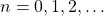? Despite the fact that the Bertrand’s (Bertrand-König’s theorem) states that the only potentials with orbits bounded and closed are the keplerian (coulombian, newtonian) force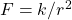with potentialand the harmonic oscillator, we can discuss the problem of periods beyond these facts (to discuss in the future!). Forwe obtain:

(32)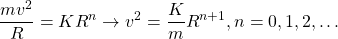so

(33)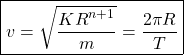(34)or

(35)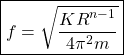With the same techniques, we can even derive the following equations:

(36)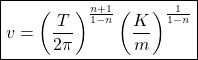or

(37)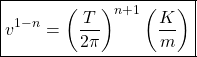You can check yourself you get for frequencies

(38)and

(39)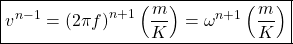Remark: take the limit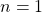to get a “classical result”.

Remark (II): the most simple gravitational waves have a frequency equal to twice the orbital frequency! Yes, I am aware of the LIGO discovery in the past year, 2016. That is one of the hidden MINOR reasons to be missing in the blogsphere.

Remark (III): You can play with the above frequencies and formulae in much more general forces. For instance, the substitution of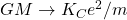reveals critical differences between the gravitational case and the electric case. Note the role of the equivalence principle!

Have you enjoyed all my extra-dimensional equations? Would you join me in my multidimensional journey?

I AM BACK! I wish I can continue posting in the next weeks/months…

This site uses Akismet to reduce spam. Learn how your comment data is processed.